Published

# Colour Detectino Based Object Tracking

I did this project to learn image processing using Open CV & Raspberry PI.

IntermediateFull instructions provided2 days250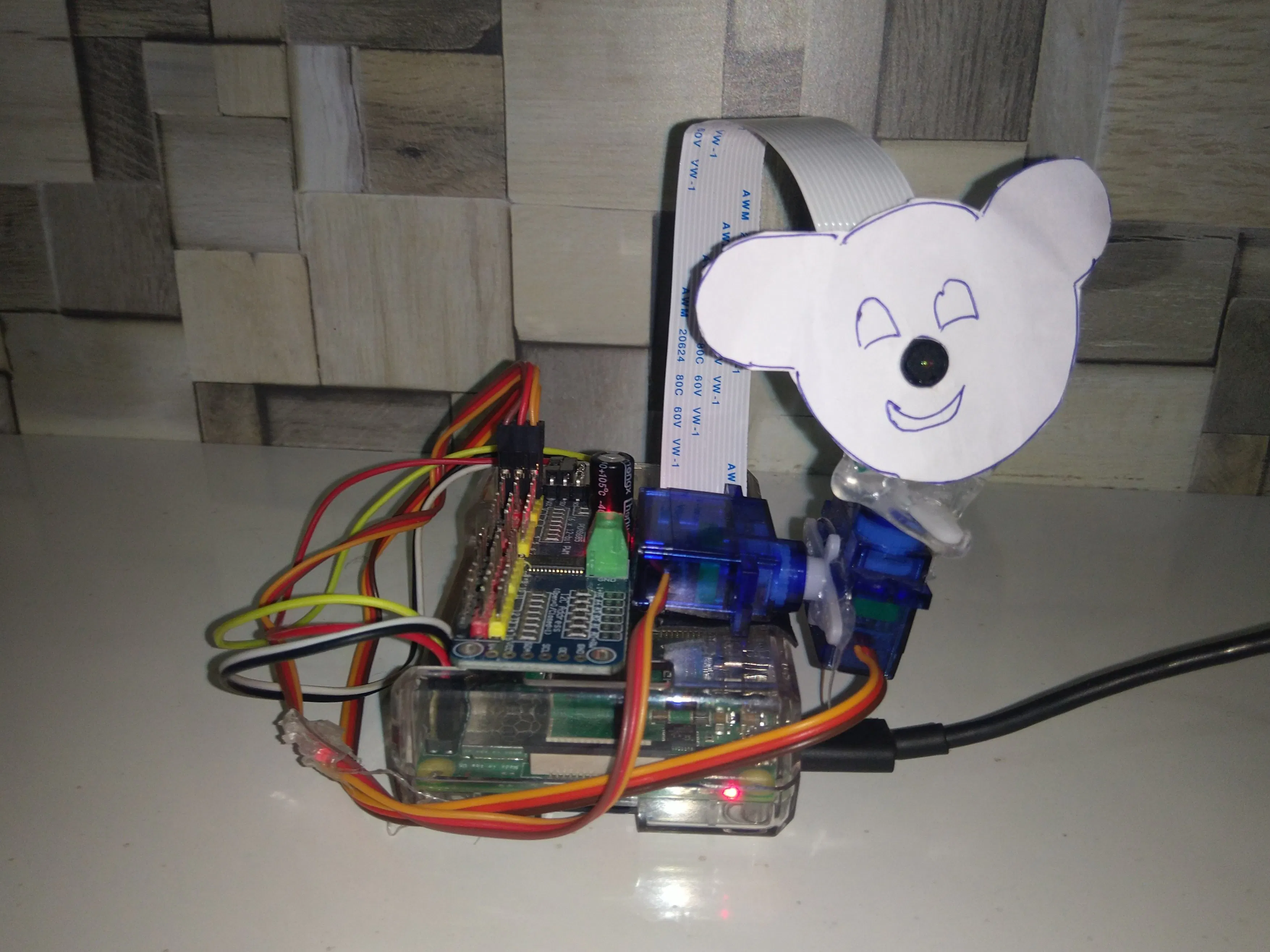## Things used in this project

### Hardware components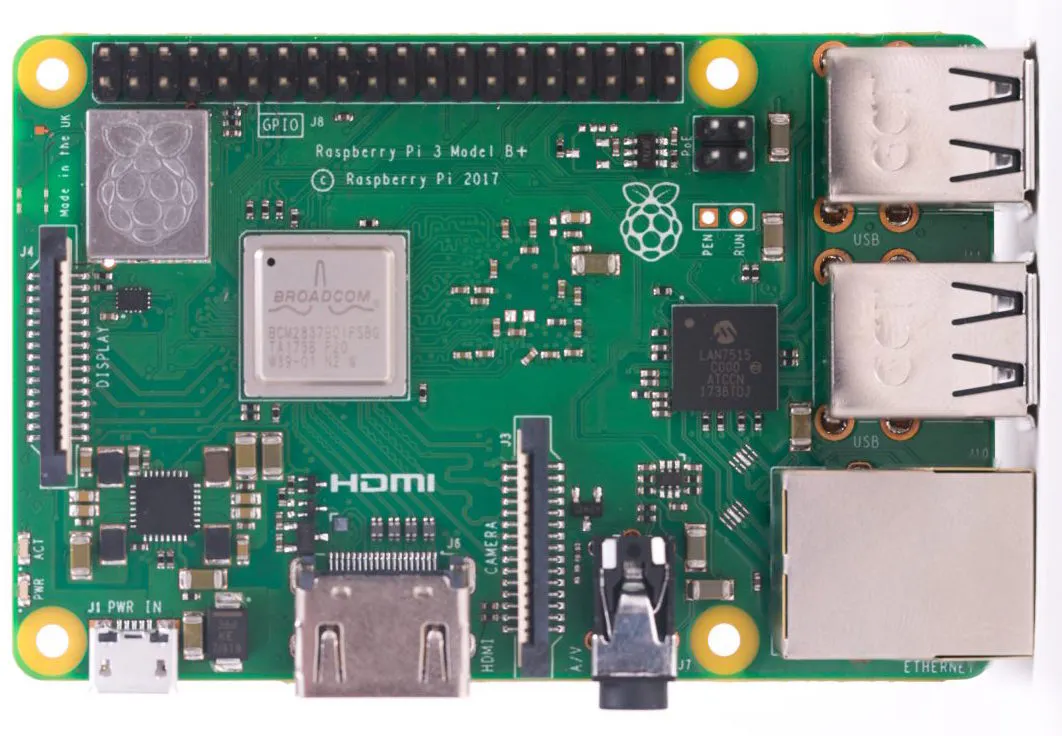Raspberry Pi 3 Model B+
×1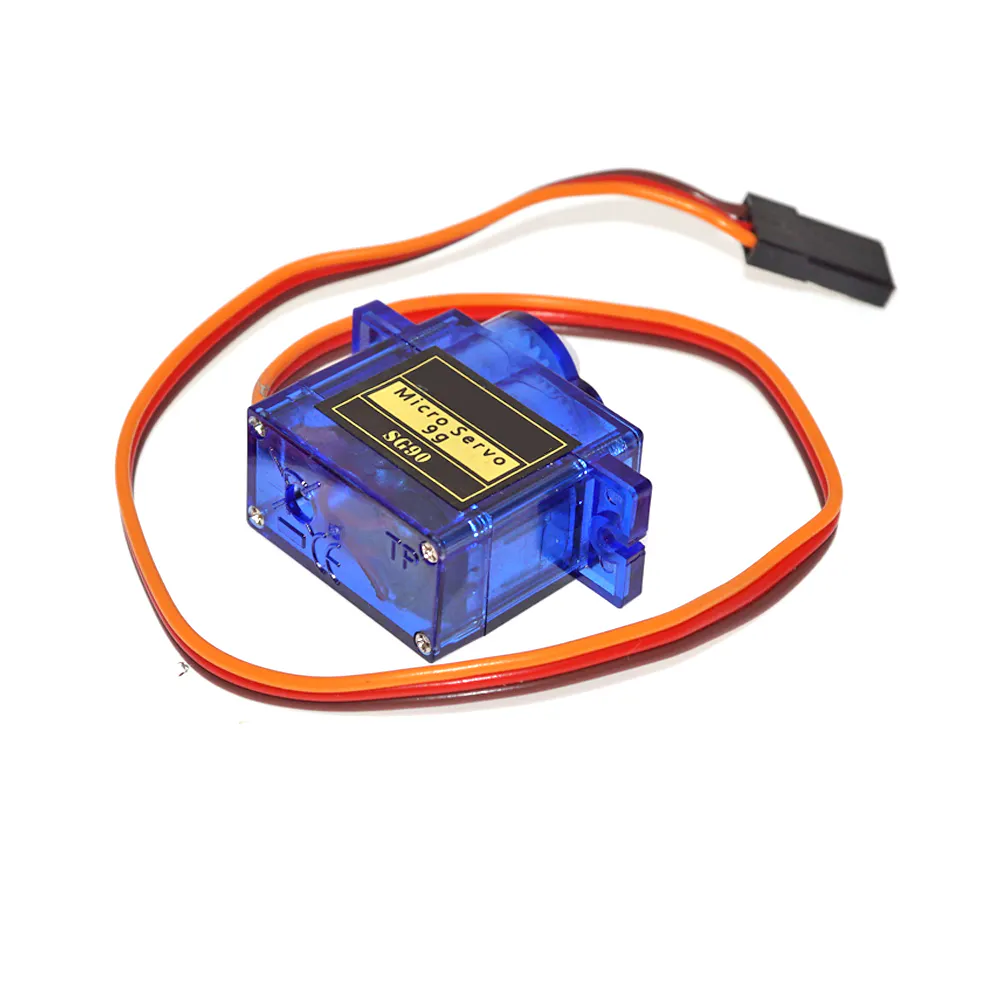SG90 Micro-servo motor
×2
 pcm 9685
×1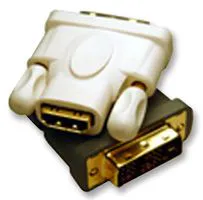DVI to HDMI Audio / Video Adapter, HDMI Receptacle
×1Jumper wires (generic)
×1

### Software apps and online servicesOpenCV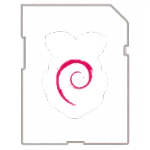Raspberry Pi Raspbian

## Schematics

### Wiring Diagram

Wiring diagram of PI, Servo Driver & Servo Motors.

## Code

### Servo position 0

Python
Check servo positon at zero
```import time
kit = ServoKit(channels=16)
kit.servo.set_pulse_width_range(650, 2610)
kit.servo.angle =(0)
kit.servo.set_pulse_width_range(650, 2610)
kit.servo.angle =(0)
```

### Servo Positon 180

Python
to check servo positon at 180 deg.
```import time
kit = ServoKit(channels=16)
kit.servo.set_pulse_width_range(900, 2610)
kit.servo.angle =(180)
kit.servo.set_pulse_width_range(900, 2610)
kit.servo.angle =(180)
```

### Servo Positon 90

Python
to check servo position at 90 deg.
```import time
kit = ServoKit(channels=16)
kit.servo.set_pulse_width_range(650, 2610)
kit.servo.set_pulse_width_range(650, 2610)
kit.servo.angle =(90)
kit.servo.angle =(90)
```

### Red_Track01

Python
Red object tracking code.
```import cv2 #Import CV2
import numpy as np #Import Numpy
from adafruit_servokit import ServoKit #Import Servo driver library
kit = ServoKit(channels=16) #Define instance of Servo library
kit.servo.set_pulse_width_range(600, 2610)   #Set Minimum & Maximum positon of X axis Servo
kit.servo.set_pulse_width_range(1102, 2170)    #Set Minimum & Maximum positon of Y axis Servo
cap = cv2.VideoCapture(0) #Catpure video from camera
Ht = 320 #Defined Height of frame
Wd = 480 #Defined Width of Frame
cap.set(3, Wd) #Set frame Width
cap.set(4, Ht) #Set frame height
_, frame = cap.read() #Store captured frame of camera to variable "frame"
rows, cols, ch = frame.shape #Get frame size
x_medium = int(cols / 2) #Initialize horizontal position
y_medium = int(rows / 2) #Initialize vertical positon

x_center = int(cols / 2) #Initialize Horizontal center position
y_center = int(rows / 2) #Initialize Vertical center position
x_position = 90 # centre posito of servo
y_position = 90 # centre posito of servo
x_band = 50
y_band = 50
#Loop
while True:
_, frame1 = cap.read() #Store Video snap in varialble "frame1"
frame2 = cv2.flip(frame1,-1) # Flip image vertically
frame2 = cv2.flip(frame1, 0) # flip image vertically
hsv_frame2 = cv2.cvtColor(frame2, cv2.COLOR_BGR2HSV)
#blurred_frame = cv2.GaussianBlur(frame1, (5, 5), 0)
#Red Colour
low_red = np.array([163,74,30]) #low HSV value for Red objects
high_red = np.array([179,255,255]) # High HSV value for Red objects
#Contors
contours_red, _ = cv2.findContours(red_mask, cv2.RETR_TREE, cv2.CHAIN_APPROX_NONE) # Findig Contours
contours = sorted(contours_red, key=lambda x:cv2.contourArea(x), reverse=True) # Arrange Contours in Assending
for cnt in contours: # Draw rectangle on First contors on image
(x,y,w,h) = cv2.boundingRect(cnt)
cv2.rectangle(frame2, (x , y) , (x + w, y + h) , (0, 255, 0), 2) # Getting Position of rectangle & line colour & thickness
break # Break loop to draw only one rectangle. if comment we get all red object rectangle
for cnt in contours:
(x,y,w,h) = cv2.boundingRect(cnt)
x_medium = int((x + x + w) / 2) # Checking horizontal center of red object & save to variable
y_medium = int((y + y + h) / 2) # Checking Vertical center of red object & save to variable
break
cv2.line(frame2, (x_medium, 0), (x_medium, Ht), (0, 255, 0), 2) #Draw horizontal centre line of red object
cv2.line(frame2, (0, y_medium), (Wd, y_medium), (0, 255, 0), 2) #Draw Vertical centre line of red object
cv2.imshow("IN Frame", frame2) #Printing frame with rectangle &  lines
# Move Horizontal Servo servo motor
if x_medium < x_center - x_band:
x_position -= 1
elif x_medium > x_center + x_band:
x_position += 1
# Move Vertiacl Servo servo motor
if y_medium < y_center - y_band:
y_position -= 1
elif y_medium > y_center + y_band:
y_position += 1
#print("x =", x_position , "y =", y_position)
if x_position >= 180:
x_position = 180
elif x_position <+ 0:
x_position = 0
else:
x_position = x_position
if y_position >= 180:
y_position = 180
elif y_position <= 0:
y_position = 0
else:
y_position = y_position
kit.servo.angle =(x_position)
kit.servo.angle =(y_position)
#Exit Key
key = cv2.waitKey(1)
if key == 27:
kit.servo.angle =(90)
kit.servo.angle =(90)
print("key", key)
break
cv2.destroyAllWindows()
cap.release()
```

### Colour value checking code

Python
this code is used to find colour filtering values.
```from imutils.video import VideoStream
from imutils.video import FPS
import imutils
import cv2
import numpy as np
def nothing(x):
pass
cap = cv2.VideoCapture(0)
cv2.namedWindow("Trackbars")
cv2.createTrackbar("test", "frame", 50, 500, nothing)
cv2.createTrackbar("color/gray", "frame", 0, 1, nothing)
cv2.namedWindow("Trackbars")
cv2.createTrackbar("L - H", "Trackbars", 0, 255, nothing)
cv2.createTrackbar("L - S", "Trackbars", 0, 255, nothing)
cv2.createTrackbar("L - V", "Trackbars", 0, 255, nothing)
cv2.createTrackbar("U - H", "Trackbars", 255, 255, nothing)
cv2.createTrackbar("U - S", "Trackbars", 255, 255, nothing)
cv2.createTrackbar("U - V", "Trackbars", 255, 255, nothing)
#Loop
while True:
_, frame1 = cap.read() #Store Video snap in varialble "frame1"
frame2 = cv2.flip(frame1,-1) # Flip image vertically
frame2 = cv2.flip(frame1, 0) # flip image vertically
frame2 = imutils.resize(frame2, width=400)
hsv_frame2 = cv2.cvtColor(frame2, cv2.COLOR_BGR2HSV)
l_h = cv2.getTrackbarPos("L - H", "Trackbars")
l_s = cv2.getTrackbarPos("L - S", "Trackbars")
l_v = cv2.getTrackbarPos("L - V", "Trackbars")
u_h = cv2.getTrackbarPos("U - H", "Trackbars")
u_s = cv2.getTrackbarPos("U - S", "Trackbars")
u_v = cv2.getTrackbarPos("U - V", "Trackbars")
blurred_frame = cv2.GaussianBlur(frame1, (5, 5), 0)
low = np.array([l_h,l_s,l_v])
high = np.array([u_h,u_s,u_v])
#Contors
contours, _ = cv2.findContours(mask, cv2.RETR_TREE, cv2.CHAIN_APPROX_NONE)
for contour in contours:
cv2.drawContours(frame2, contour, -1, (0, 255, 0), 2)
#Printing frames
cv2.imshow("IN Frame", frame2)